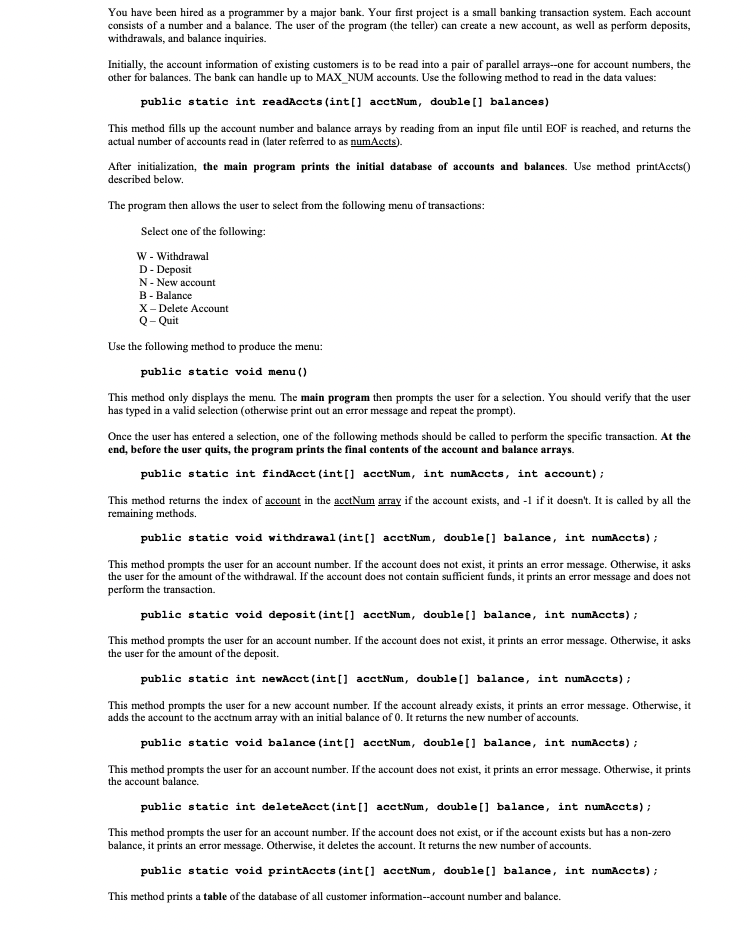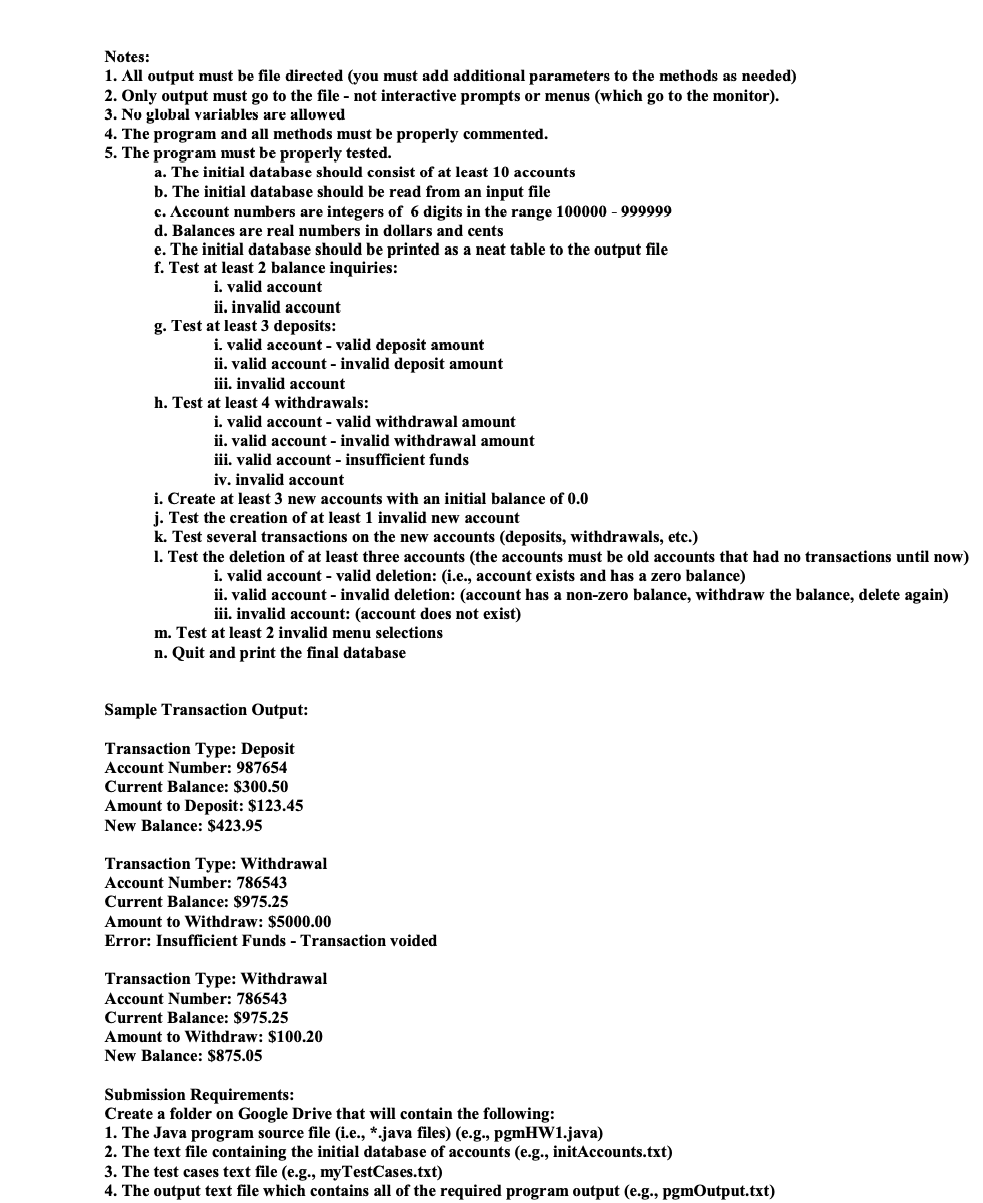Home / Expert Answers / Computer Science / java-programjava-you-have-been-hired-as-a-programmer-by-a-major-bank-your-first-project-is-a-small-pa444

# (Solved): JAVA PROGRAMJAVA You have been hired as a programmer by a major bank. Your first project is a small ...

JAVA PROGRAMJAVAYou have been hired as a programmer by a major bank. Your first project is a small banking transaction system. Each account consists of a number and a balance. The user of the program (the teller) can create a new account, as well as perform deposits, withdrawals, and balance inquiries. Initially, the account information of existing customers is to be read into a pair of parallel arrays-one for account numbers, the other for balances. The bank can handle up to MAX_NUM accounts. Use the following method to read in the data values: public static int readAccts (int[] acctNum, double[] balances) This method fills up the account number and balance arrays by reading from an input file until EOF is reached, and returns the actual number of accounts read in (later referred to as numAccts). After initialization, the main program prints the initial database of accounts and balances. Use method printAccts 0 described below. The program then allows the user to select from the following menu of transactions: Select one of the following: W - Withdrawal D - Deposit N - New account B - Balance X - Delete Account Q - Quit Use the following method to produce the menu: public static void menu() This method only displays the menu. The main program then prompts the user for a selection. You should verify that the user has typed in a valid selection (otherwise print out an error message and repeat the prompt). Once the user has entered a selection, one of the following methods should be called to perform the specific transaction. At the end, before the user quits, the program prints the final contents of the account and balance arrays. public static int findAcct(int[] acctNum, int numAccts, int account); This method returns the index of account in the acctNum array if the account exists, and $$-1$$ if it doesn't. It is called by all the remaining methods. public static void withdrawal (int[] acctNum, double[] balance, int numAccts); This method prompts the user for an account number. If the account does not exist, it prints an error message. Otherwise, it asks the user for the amount of the withdrawal. If the account does not contain sufficient funds, it prints an error message and does not perform the transaction. public static void deposit(int[] acctNum, double[] balance, int numAccts); This method prompts the user for an account number. If the account does not exist, it prints an error message. Otherwise, it asks the user for the amount of the deposit. public static int newAcct(int[] acctNum, double[] balance, int numAccts); This method prompts the user for a new account number. If the account already exists, it prints an error message. Otherwise, it adds the account to the acctnum array with an initial balance of 0 . It returns the new number of accounts. public static void balance (int[] acctNum, double[] balance, int numAccts); This method prompts the user for an account number. If the account does not exist, it prints an error message. Otherwise, it prints the account balance. public static int deleteAcct(int[] acctNum, double[] balance, int numAccts); This method prompts the user for an account number. If the account does not exist, or if the account exists but has a non-zero balance, it prints an error message. Otherwise, it deletes the account. It returns the new number of accounts. public static void printAccts (int[] acctNum, double[] balance, int numAccts); This method prints a table of the database of all customer information-account number and balance. Notes: 1. All output must be file directed (you must add additional parameters to the methods as needed) 2. Only output must go to the file - not interactive prompts or menus (which go to the monitor). 3. No global variables are allowed 4. The program and all methods must be properly commented. 5. The program must be properly tested. a. The initial database should consist of at least 10 accounts b. The initial database should be read from an input file c. $$\Lambda c c o u n t$$ numbers are integers of 6 digits in the range $$100000-999999$$ d. Balances are real numbers in dollars and cents e. The initial database should be printed as a neat table to the output file f. Test at least 2 balance inquiries: i. valid account ii. invalid account g. Test at least 3 deposits: i. valid account - valid deposit amount ii. valid account - invalid deposit amount iii. invalid account h. Test at least 4 withdrawals: i. valid account - valid withdrawal amount ii. valid account - invalid withdrawal amount iii. valid account - insufficient funds iv. invalid account i. Create at least 3 new accounts with an initial balance of $$0.0$$ j. Test the creation of at least 1 invalid new account $$k$$. Test several transactions on the new accounts (deposits, withdrawals, etc.) l. Test the deletion of at least three accounts (the accounts must be old accounts that had no transactions until now) i. valid account - valid deletion: (i.e., account exists and has a zero balance) ii. valid account - invalid deletion: (account has a non-zero balance, withdraw the balance, delete again) iii. invalid account: (account does not exist) $$\mathrm{m}$$. Test at least 2 invalid menu selections n. Quit and print the final database Sample Transaction Output: Transaction Type: Deposit Account Number: 987654 Current Balance: $$\mathbf{\ 3 0 0 . 5 0}$$ Amount to Deposit: $$\ 123.45$$ New Balance: $$\mathbf{\ 4 2 3 . 9 5}$$ Transaction Type: Withdrawal Account Number: 786543 Current Balance: $$\ 975.25$$ Amount to Withdraw: $$\ \mathbf{\ 5 0 0 0 0}$$ Error: Insufficient Funds - Transaction voided Transaction Type: Withdrawal Account Number: 786543 Current Balance: $$\ 975.25$$ Amount to Withdraw: $$\mathbf{\ 1 0 0 . 2 0}$$ New Balance: $$\ 875.05$$ Submission Requirements: Create a folder on Google Drive that will contain the following: 1. The Java program source file (i.e., *.java files) (e.g., pgmHW1.java) 2. The text file containing the initial database of accounts (e.g., initAccounts.txt) 3. The test cases text file (e.g., myTestCases.txt) 4. The output text file which contains all of the required program output (e.g., pgmOutput.txt)

We have an Answer from Expert